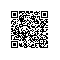# 如何阅读框架源代码## 二、了解框架的设计思想

    //这个方法可以获得point2顶角的弧度值
function getAngle(point1, point2, point3) {
var bb = (point2.y - point1.y)*(point2.y - point1.y) + (point2.x - point1.x)*(point2.x - point1.x);
var aa = (point3.y - point1.y)*(point3.y - point1.y) + (point3.x - point1.x)*(point3.x - point1.x);
var cc = (point3.y - point2.y)*(point3.y - point2.y) + (point3.x - point2.x)*(point3.x - point2.x);
var cosa = (bb + cc - aa)/(2*Math.sqrt(bb)*Math.sqrt(cc));
return Math.acos(cosa);
}

getAngle方法接收三个坐标参数，可以计算出 point2 顶角的弧度值，如果不告诉你这使用了运用三角形的余弦定理，恐怕你看半天也看不出来这是怎么算出来的

• 到框架的官网看文档，开源的框架，甭管详不详细，这肯定是第一手权威资料
• 到网上搜别人的分析总结(源码分析也不会少)，大多数情况下，都会有大神已经研究过了，站在巨人的肩膀上，能省不少事

## 五、坚持不放弃使用钉钉扫一扫加入圈子
+ 订阅